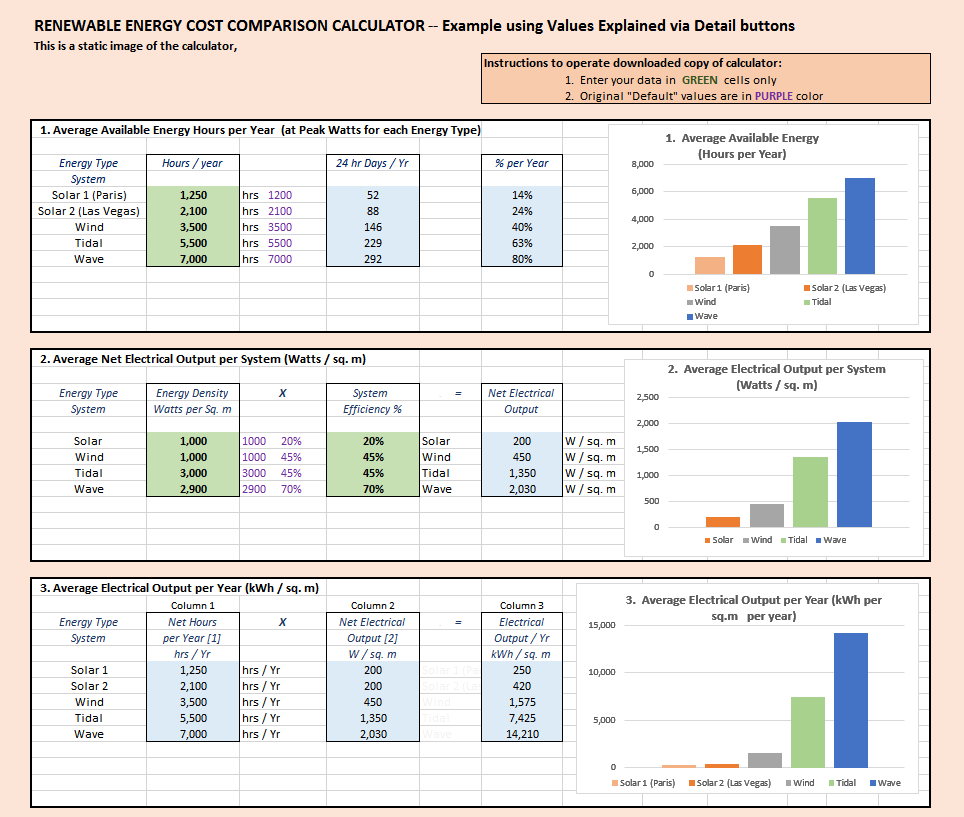Tidal Flow Turbine System COST                Assumptions

Based on our discussions with tidal and river turbine manufacturers we have determined that the cost to produce, deploy and tie into the grid an 18 kW turbine with a 5 m diameter turbine to be 100,000 USD.

hence 5m dia = 20 sq. m = 5,000 USD / sq. m

International Renewable Energy Agency (IRENA)

TIDAL ENERGY TECHNOLOGY BRIEF   (June 2014)  Reports:

Then, according to our calculation example tidal output per year per sq. m = 5,400 kWh X 20 yrs =108,000 kWh at \$.27 / kwh  X 108,000 = \$29,160 / sq. m for tidal  turbine systems.

Therefore a 5 m diameter turbine system would cost  = 20 sq. m x \$29,160 = \$583,200 which seems a little high even for a custom made system.

However if the deployment were in the Bay of Fundy  with a 3.3 m / sec flow then the income per sq. m at \$.15 / kWh over 20 years would be \$ 4,860 x 20 yrs =  \$97,200  less 29,160 cost for a gross profit of \$ 68,000  (after 20 years)

so perhaps this is actually not a very high cost estimate after all.

see Tidal Density Assumptions

For the calculator example we use a Tidal Turbine installed cost of  \$5,000 per sq. m

Tidal current technologies are still in the demonstration stage, so cost estimates are projected to decrease with deployment.
Estimates from across a number of European studies for 2020 for current tidal technologies are between EUR 017/kWh and EUR 0.23/kWh, although current demonstration projects suggest the levelised cost of energy (LCOE) to be in the range of EUR 0.25-0.47/kWh.

It is important to note that costs should not be considered as a single performance indicator for tidal energy.

For example, the costs for both tidal range and tidal stream technologies can fall by up to 40% in cases where they are combined and integrated in the design and construction of existing or new infrastructure.

hence:    average price 17 + 23 = 40/2= 20 EUR
(June 2014) convert to USD at 1.3691 EUR = 0.27 USD /kWh (assuming this is over 20 years)

Click X  to close this note:

NOTE:
The Cost Value used in the calculator example is assumed to be the capital cost to obtain and install the system to grid tie in.
The rest of the calculations evaluate each system against this cost metric only.

The calculator does not accomodate mortgage payments to pay off loans, or costs for land rent if required (aka a fuel cost for renewables), or operations and maintenance costs, or any other ongoing or futre costs associated with the energy production unit.

Of course you can put any cost value you want in the downloaded copy of calculator and modify other parts of the calculator to suit your needs, or even modify the calculator.

2010+     2015           2016+        Comparisons